# Multi-class hexbins

Hexagonal binning (proposed by Dan Carr and co-workers in 1987) is a standard approach to the display of large two-dimensional numeric data sets. Compared to scatterplots with alpha-blending based on full pixel-resolution binning it has lower spatial resolution but produces output that can be stored more compactly and rendered more rapidly. Compared to the implementations of alpha-blending based on overplotting and an 8-bit alpha channel, as with `plot()` in R, it allows for much larger data sets.

An important limitation of hexagonally binned scatterplots is that they do not allow for the display of multi-class data using colours in a single graph panel; faceting is necessary. The `hextri` package allows the use of colour for multi-class data by dividing each hex into six triangles and allocating these in proportion to the number of observations from each class in the hex. In fact, the package allows for observation weights, so the allocation is actually in proportion to the total weight for each class in the hex. The package implements two styles of hexbin plot that display the total hex size differently: `alpha`makes the opacity of each hex proportional to the total weight for the hex, `size` makes the area of the hex proportional to the total weight.

### An example: air pollution

Here’s an example using the data on 1973 New York summer air pollution in the `airquality` data set: a graph of temperature by solar radiation with ozone concentration (smog) as the class variable. At the moment, the package doesn’t handle missing data well, so we run `na.omit()` first.

``````library(hextri)
data(airquality)
airquality\$o3group<-with(airquality, cut(Ozone, c(0,18,60,Inf)))

with(na.omit(airquality),
hextri(Solar.R, Temp, class=o3group, colours=c("skyblue","grey60","goldenrod"), style="size",
)``````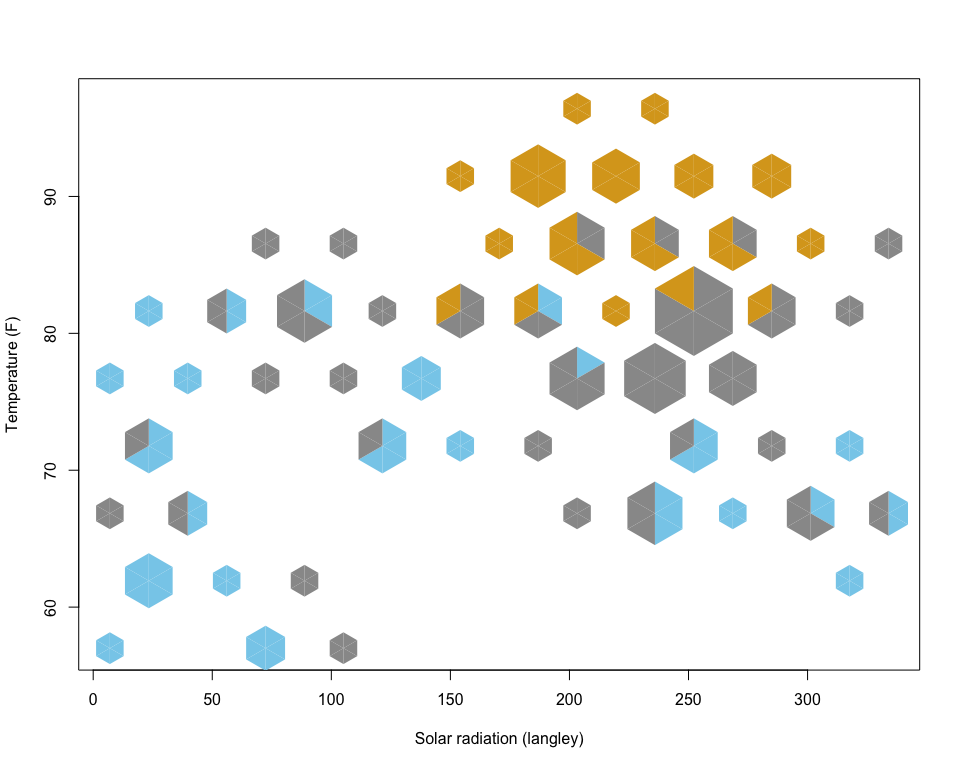The graph shows the non-monotone relationship of temperature and solar radiation, and the tendency for smog to appear on hot, sunny days.

Tthe `lattice` package allows for the plot to be conditioned on wind speed, the other important variable

``````library(lattice)
xyplot(Temp~Solar.R|equal.count(Wind,4), groups=o3group, panel=panel.hextri,
data=na.omit(airquality), colours=c("royalblue","grey60","goldenrod"),
strip=strip.custom(var.name="Wind Speed"),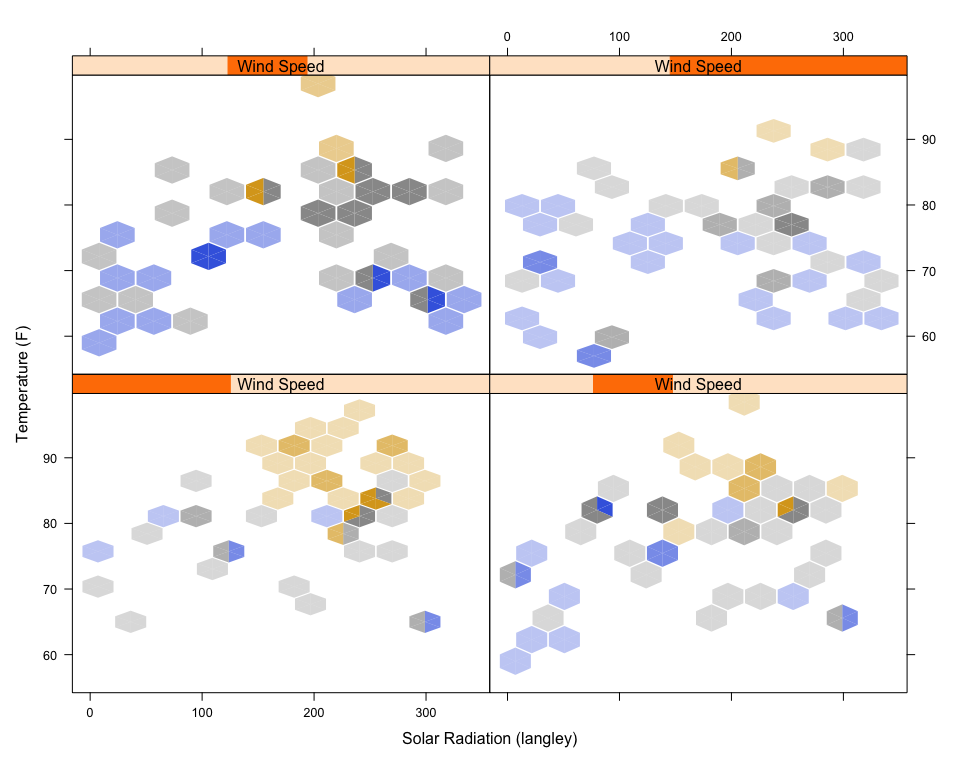High wind days tend not to be as hot, but also tend to have less ozone than would otherwise be expected.

### Another example: Iron in NHANES

The `hexbin` package has a moderately large dataset from the National Health And Nutrition Examination Surveys (NHANES), looking at various measures of iron availability.

A fairly dramatic one is haemoglobin by age, tabulated by sex (orange for women, purple for men). The first plot uses alpha-blending to depict total hex size. The increase in size at age 65 is due to oversampling of older people.

``````data(NHANES, package="hexbin")
with(na.omit(NHANES[,-8]), hextri(Age,Hemoglobin, class=Sex,colour=c("orange","purple"),
nbins=20,xlab="Age",ylab="Serum haemoglobin"))``````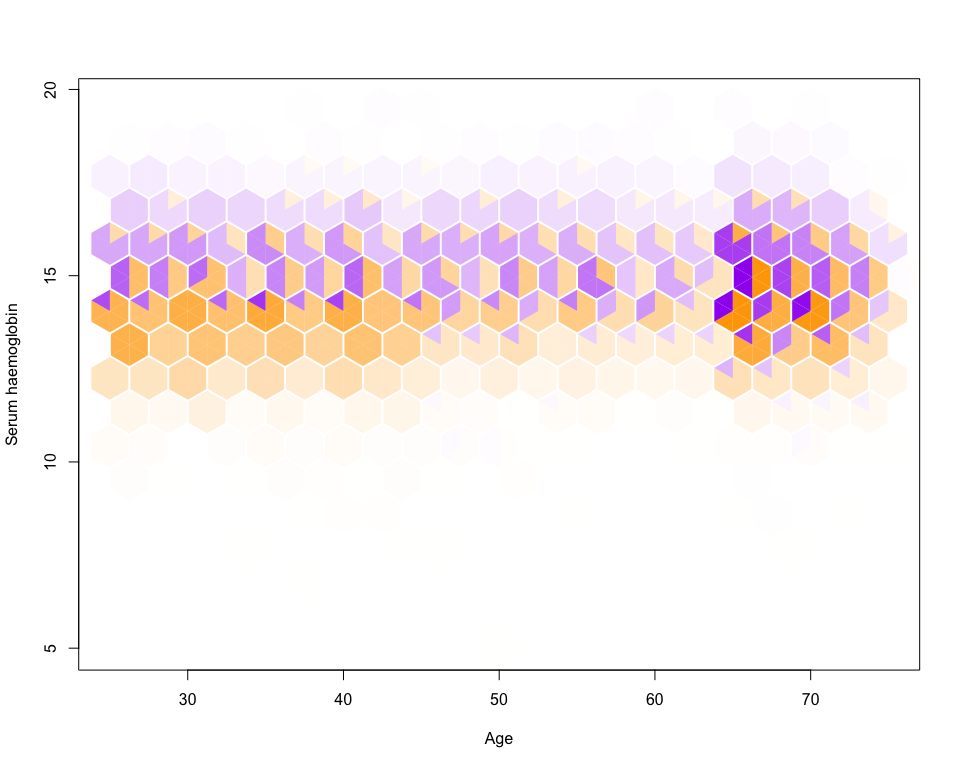Parts of this graph show one of the problems with a regular assignment of triangles within the hex: three-dimensional artifacts because a cube seen corner-on is a hexagon. This next plot has random ordering of triangles within each hex. It also has diffusion of rounding error: when the class proportions within a hex do not divide equally into sixths, the rounding error is divided up among nearby hexes that have not yet been rendered. Error diffusion is especially important with small classes, as otherwise a class with less than 1/12 of the total sample would never be seen.

``````with(na.omit(NHANES[,-8]), hextri(Age,Hemoglobin, class=Sex,colour=c("orange","purple"),
nbins=20,xlab="Age",ylab="Serum haemoglobin", diffuse=TRUE))``````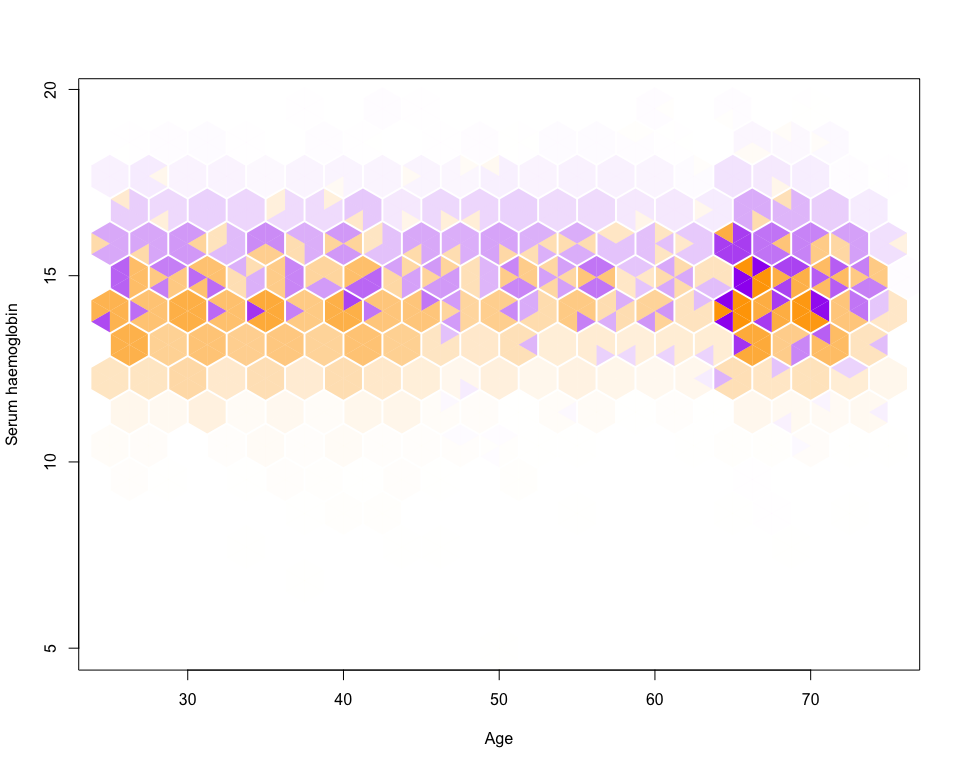Here is the same graph with hex totals depicted by size, with and without error diffusion

``````with(na.omit(NHANES[,-8]), hextri(Age,Hemoglobin, class=Sex,colour=c("orange","purple"),
nbins=20,xlab="Age",ylab="Serum haemoglobin",style="size"))``````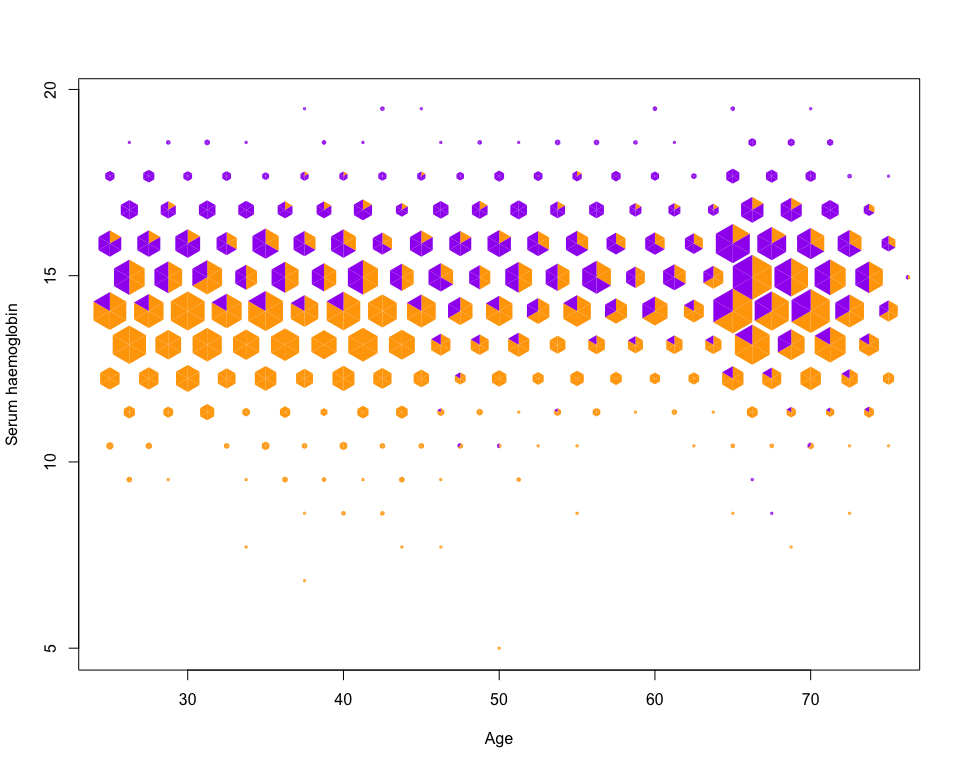``````with(na.omit(NHANES[,-8]), hextri(Age,Hemoglobin, class=Sex,colour=c("orange","purple"),
nbins=20,xlab="Age",ylab="Serum haemoglobin", diffuse=TRUE,style="size"))``````